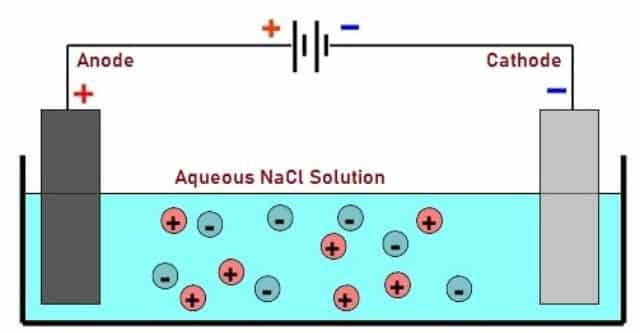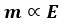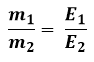Thursday, March 30, 2023
HomeFaradays Laws of ElectrolysisFaraday Laws of Electrolysis - First Law, Second Law and its Applications

# Faraday Laws of Electrolysis – First Law, Second Law and its Applications

Faraday Laws of Electrolysis are the fundamental laws that describes the significance of electrolytic effects. This post will discuss what is Electrolysis, What are the Faraday Laws of Electrolysis (First & Second Law), its applications, advantages and disadvantages.

Michael Faraday is known for his contributions in the field of Electrochemistry. He discovered the two laws of Electrolysis which states that the amount of chemical change or decomposition is exactly proportional to the quantity of Electricity that is passed in the solution.Fig. 1 – Introduction to Faraday Laws of Electrolysis

During this chemical change (Oxidation or Reduction) the amount of substance that is deposited or dissolved are proportional to their chemical equivalent weights. The amount of charge required to achieve this is equal to charge on one mole of electrons known as 1 Faraday which is equal to 96,500 Coulombs. The laws basically state the quantitative aspects of Electrolysis based on the electrochemical research published by Michael Faraday in the year 1833.Fig. 2 – Image of Michael Faraday

## What is Electrolysis

The process in which Electrical energy causes non-spontaneous chemical reaction is known as Electrolysis. Electrolysis forms the basis for Electrosynthesis of chemical compounds. In this process, chemical changes occur through reactions at electrodes which comes in contact with an electrolyte when electric current is passed through it.

During this process, positively charged ions drift towards the negative electrode and negatively charged ions drift towards the positive electrode. Positively charged ions receive electrons whereas negatively charged ions lose electrons. Oxidation occurs at anode and Reduction occurs at Cathode.

Let us consider Fig. 3, where the Electrodes are dipped into aqueous solution of Sodium Chloride. When Electricity is passed through Sodium Chloride solution, Hydrogen gas is produced at the negative electrode and Chloride gas is oxidized to Chlorine at positive electrode.Fig. 3 – Electrolysis of Sodium Chloride

The two laws of Electrolysis are:

• Faraday First Law of Electrolysis
• Faraday Second Law of Electrolysis

### Faraday First Law of Electrolysis

The First law in Faraday Laws of Electrolysis states that, “The amount of chemical reaction occurring at any electrode due to the effect of electrical energy is directly proportional to the quantity of electricity passed through the electrolyte”.

Let us consider the amount of substance deposited or dissolved at electrodes is ‘m’ and ‘Q’ is the quantity of charge (electricity) passed through the electrolyte. According to Faraday First Law:where, Z = Electrochemical Equivalent

The relation between Chemical Equivalent (E), Faraday Constant (F) and Electrochemical Equivalent (Z) is defined by the equation:We Know that, Q = I x t

Where,

• Q = Quantity of Charge (electricity) in Coulombs (Q),
• I = Current in Amperes (A),
• t = Time (seconds)

Hence, the equation m = ZQ can also be written as:### Faraday Second Law of Electrolysis

The Second Law in Faraday Laws of Electrolysis states that – “The mass of the substance that gets deposited when the same quantity of charge (electricity) is passed through the electrolytic solution is directly proportional to their chemical equivalent weights”.

This law can be represented mathematically as:where,

• m = Mass of the Substance
• E = Equivalent Weight of the Substance

It can also be expressed as:Equivalent weight or chemical equivalent of a substance can be defined as the ratio of its weight and valency.## Applications of Faraday Laws of Electrolysis

The applications of Faraday Laws of Electrolysis include:

• Faraday laws helps in the determination of equivalent masses of elements.
• Based on the Laws of Electrolysis, non-metals like Hydrogen, Fluorine, chlorine are obtained.
• They are also applied in the field of Electron Metallurgy for obtaining metals like sodium, potassium.
• The process of Electroplating involves Electrolysis.
• Electro-refining of metals is carried out by Electrolysis.
• Electrolysis is an answer to renewable electricity storage.Fig. 4 – Copper Electrolysis Refining Plant

• Industrial chemicals are easily produced with Electrolysis process.
• The process is efficient and cost effective.

• Energy required is high.
• Electrolysis based industries produces large amounts of effluents as wastes.
• Wastes from these plants contribute to environmental pollution.
```Also Read:
De Morgan’s Laws – First and Second Law, Verification and Applications
Electric Field – Gauss’s & Coulomb’s Law, Electric Field Lines, Applications
Ohms Law – Voltage, Current & Resistance Relation, When Not Applicable
Kirchhoff’s Laws of Current & Voltage – Application, Advantage, Limitation```Laxmi Ashrit
Laxmi is a B.E (Electronics & Communication) and has work experience in RelQ Software as Test Engineer and HP as Technical support executive. She is an author, editor and partner at Electricalfundablog.
RELATED ARTICLES